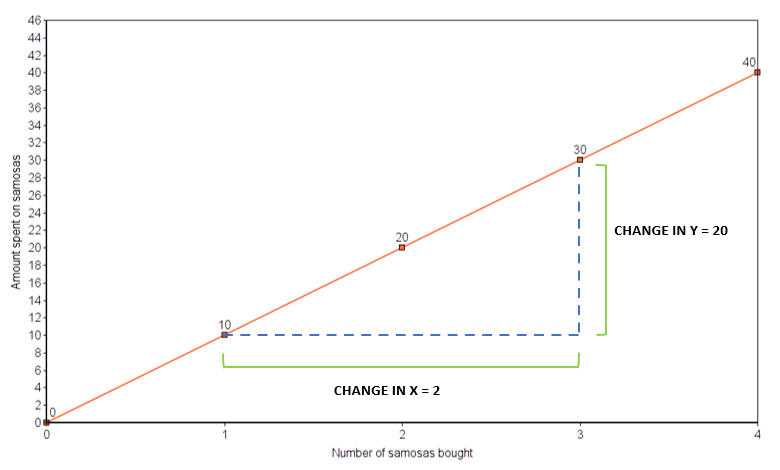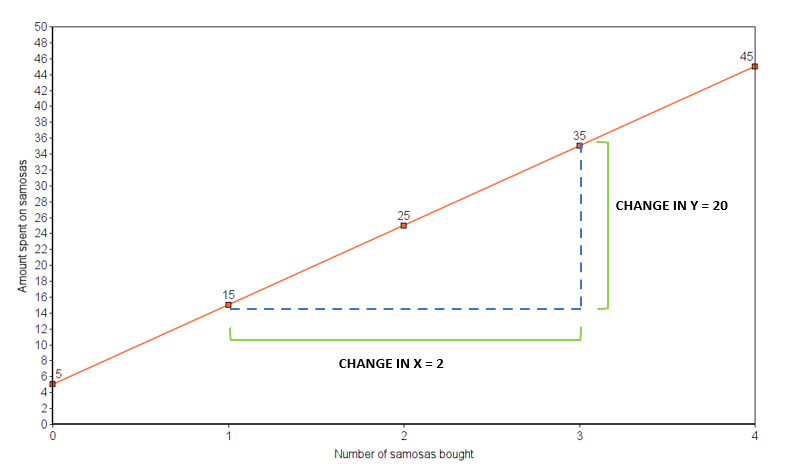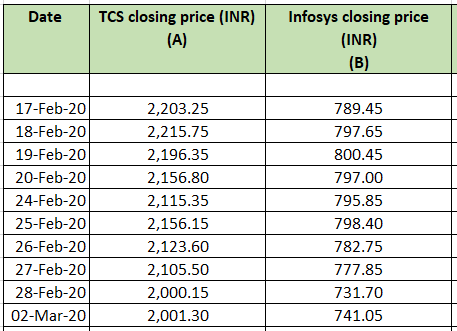# Straight line equation method

4.1Up until now, you’ve seen how it’s possible to trade in pairs by tracking two correlated shares and making use of a temporary weakening in their relationship. The variables we saw were differentials, spreads and price ratios. While that is one way to track pairs, there’s another method to trading in pairs too.

Like the first method, this one also involves understanding the relationship between two stocks. But here, we consider one stock as a dependent asset, while the other is independent. In other words, the movement of one stock can be studied in relation to the movement of the other. It’s for this reason that we call this method as Relative Value Trading (RVT).

That’s a very basic and concise way to explain what this second method is all about. But to get into the details, we’ll once again need to rewind a bit and revisit some more high school math. So, let’s get started.

### The straight line equation

To jump right into it, you may perhaps recall learning how to find the equation of a straight line in school. It goes something like this:

y = mx + c

Rings a bell? Well, whether you recall it vaguely or whether you have no memory of hearing this in school, don’t worry. This chapter will help you catch up on the straight line equation, which is the first step to understanding the RVT method.

Now, what do the letters x, y, m and c in the equation mean?

Perhaps you already have a fair idea of what x and y are. They are the variables that we’ll be considering. One of these is a dependent variable, while the other is independent.

• x is the independent variable here
• y is the dependent variable, since its value depends on x

Want to understand this better? Let’s look at a simple analogy to understand dependent and independent variables.

### Dependent and independent variables: An elementary example

Let’s construct a hypothetical scenario to understand dependent and independent variables. Say there’s a local food joint near your home. You head there every Saturday evening for a quick snack. And each weekend, you have your favourite food item - samosas.

Some weekends, you have just one. On others, depending on your mood, you may have more. Now, depending on how many samosas you buy, the amount you spend at the food joint varies. Let’s say each samosa costs Rs. 10. Then, here’s what your expenses may look like depending on the number of samosas you have.

 Weekend number Number of samosas bought  (A) Rate per samosa (B) Total amount spent  (A x B) Weekend 1 2 Rs. 10 Rs. 20 Weekend 2 1 Rs. 10 Rs. 10 Weekend 3 4 Rs. 10 Rs. 40 Weekend 4 3 Rs. 10 Rs. 30

You probably know where this is heading. The total amount you spend depends on the number of samosas you eat.

• So, the number of samosas you have is the independent variable (x).
• And the amount you spend is the dependent variable (y).

### Decoding the straight line equation: The slope/gradient

Recall the straight line equation. There was one variable - m. Remember?

What does that represent?

Mathematically speaking, the letter m in the equation denotes the slope or the gradient. It’s called the slope because when you represent the two variables on a graph, the slope of the line is what ‘m’ is.

The formula for the slope goes like this:

 Slope = (Change in y) ÷ (Change in x)

To understand this better, let’s plot the data from the table above on a graph.So, the slope here is 20 ÷ 2, which is 10.

What is the significance of the slope or the gradient? Surely, it’s much more than merely the slope of a graphical line, right? If that’s what you’re wondering, well, let’s see what the gradient signifies. It represents the rate of change in the dependent variable, for each unit change in the independent variable.

In this case, for each additional samosa you buy, your total expense goes up by 10 (which is the gradient). See how it fits together?

Now, there’s just one more part of the equation you need to understand. Remember the letter c that featured in the straight line equation. It represents the intercept. What is that? Let’s begin by looking at a slight modification to this example to understand what the last remaining piece of the equation is.

# The intercept: An elementary example

Take the same hypothetical scenario from above. Your local food joint. Your weekend samosa binge. All that remains the same. There’s just one minor addition in this iteration. Let’s assume that in addition to paying for the samosas you buy, you also tip the serviceperson each time. For the sake of maintaining a reasonable scale, let’s say you tip them Rs. 5 each time, irrespective of how many samosas you buy.

In this case, here’s how the data for your total expense will look like.

 Weekend number Number of samosas bought  (A) Rate per samosa (B) Tip amount  (C) Total amount spent  (A x B) + C Weekend 1 2 Rs. 10 Rs. 5 Rs. 25 Weekend 2 1 Rs. 10 Rs. 5 Rs. 15 Weekend 3 4 Rs. 10 Rs. 5 Rs. 45 Weekend 4 3 Rs. 10 Rs. 5 Rs. 35

The graph for this new set of data looks like this.See how the slope remains the same. The only thing that’s different is the point where the line cuts the y axis. In the previous case, it cut the y axis at 0.

Now, it cuts the y axis at 5.

This is the effect of the additional tip of Rs. 5 that remained constant across all your transactions. And this is what the ‘c’ in the straight line equation is all about. It is the intercept, and it is always a constant.

Basically, it is the point where the line intercepts the y axis.

### Putting the straight line equation together

Based on the data we’ve taken in the example above, here’s what we have.

• The dependent variable (y) = The amount spent at the food joint
• The independent variable (x) = The number of samosas bought
• The intercept = The tip of Rs. 5

So, the straight line equation in this case is:

 y = 10x + 5

### Working with stock price data

Up until now, we’ve taken up a very elementary example. But when you apply this to stock prices, here’s a preview of what the data may look like.Now, how do you find out the gradient and the intercept for this set of observations? It’s not possible to compute this by merely glancing through the data. You may argue that we can simply plot these points on a graph and identify the slope and the intercept.

You’re right. That’s possible.

However, that may seem doable for this particular set of observations, since there are only around 10 values for each stock price. But if you’re analysing a month’s worth of prices, or one year’s set of prices, plotting it on a graph becomes tedious and impractical.

What’s the solution then? Linear regression. And that’s what we’ll be looking at in the next chapter.

#### Wrapping up

This chapter was a basic introduction to the straight line equation. To understand how to apply it to trading, we’ll need to explore a few more concepts. Head to the next chapter to find out the details.

#### A quick recap

• The relative value trading method can also be used for pair trading.
• It begins with an understanding of the straight line equation.
• The equation goes like this: y = mx + c
• Here, y is the dependent variable while x is the independent variable.
• The ‘m’ represents the slope or the gradient. In other words, it shows the rate of change in y for one unit change in x.
• The ‘c’ represents the intercept, or the point where the graphical line cuts the y axis.
• The intercept is always a constant.